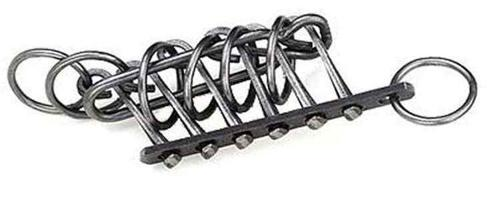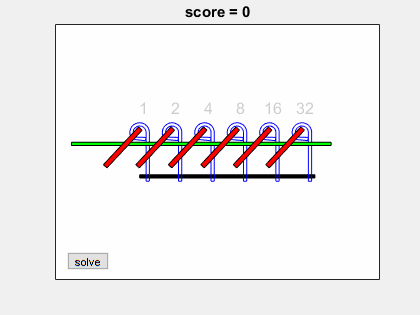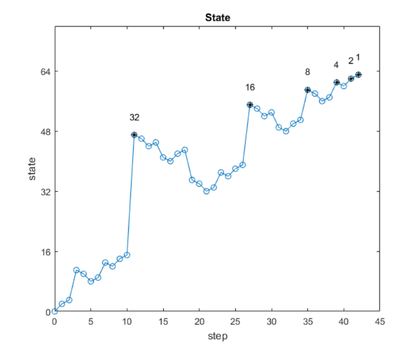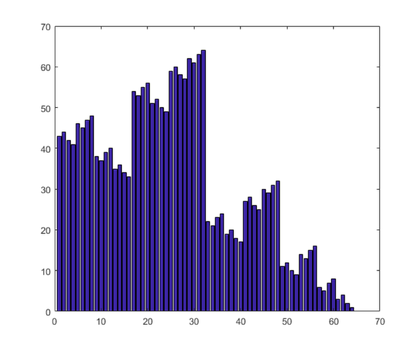# Patience Chinese Rings Puzzle

MIT's Professor Daniel Frey recently introduced me to an ancient mechanical puzzle known as "Chinese Rings", "Patience", or "Baguenaudier." I have modified Daniel's simulator to produce a new app. The state space of the puzzle forms a hypercube.### Contents

#### Background

There are dozens of YouTube videos showing solutions and speed contests for the tavern puzzle called "Patience". The best video is by Ty Yann, "Baguenaudier Chinese Rings." Here is the link to his puzzle tutorial.

Wikipedia tell us that Baguenaudier is French for "time-waster".

Prof. Frey has written a nice MATLAB simulator for his course this spring at MIT, 2.086, "Numerical Computation for Mechanical Engineers." My new app is based on his program.

#### Puzzle

The puzzle has six steel hooks, often made from nails, attached to a base. A steel ring is attached to each hook. A long movable piece known as the shuttle is initially threaded around the nails and through the rings. The objective is to free the shuttle. It ain't easy.Image credit: Daniel Frey, MIT

My new interactive app, patience, is included with version 2.2 of Cleve's Laboratory in MATLAB Central. I hope you will try to solve the puzzle yourself before you resort to the "no patience" automated solve button. The following animated gif shows several steps at the start of the solution, then skips many intermediate steps before showing the last several.#### State

The position of the six hooks, up or down, provides a six-bit binary number that characterizes the state of the mechanism. Initially, the rings are all engaged, so the state in binary is 000000, which, of course, is a decimal 0. The objective is to free all the rings, giving a binary 111111, or decimal 63. The app shows the decimal value at each step in the figure title.

The hooks are numbered from 1 through 6 starting on the left. So, we will use "big endian" binary, with the least significant bit at the left.

#### Rule

This one line in patience.m, which refers to the state vector S and a hook number n, controls the possible moves.

   dbtype 52:53 patience

52            % Is the move allowed ?
53            ok = (n==1) || (n==2)&&~S(1) || (n>2)&&(~S(n-1)&&all(S(1:n-2)));


The rule says

• Hook 1 can always be toggled.
• Hook 2 can be toggled if hook 1 is up.
• Any hook numbered 3 through 6 can be toggled if the hook immediately to its left is up and all the others before it are down.

That's all you need to know to operate the puzzle.

It turns out that at any step at most two, and sometimes only one, of the six hooks is a legal move.

For example, if the state is

S = [1 1 0 0 1 0]


there are two possible moves. You can click on hook 1 to give

S = [0 1 0 0 1 0]


Or, you can click on hook 4 to give

S = [1 1 0 1 1 0]


#### Shortest Path

The optimum path from a given starting state, such as 0, to the goal, 63, can be found by a brute force recursive search that tries all possible moves at each step. The code is at the end of this post. Here is the shortest path from 0 to 63.

   path = shortest_path(0)

path =
Columns 1 through 13
0     2     3    11    10     8     9    13    12    14    15    47    46
Columns 14 through 26
44    45    41    40    42    43    35    34    32    33    37    36    38
Columns 27 through 39
39    55    54    52    53    49    48    50    51    59    58    56    57
Columns 40 through 43
61    60    62    63


Notice that a shortest path never repeats any state because if we find ourselves reaching a state that we've already visited, we could shorten that path by skipping the extra steps.

It turns out that the only starting state that visits all vertices is 31 = [1 1 1 1 1 0], which corresponds to starting with the sixth hook up and all the others down.

Each step changes the state by a power of two. You can recover the sequence of mouse clicks that would generate this path by taking the log2 of the changes.

   hooks = log2(abs(diff(path))) + 1

hooks =
Columns 1 through 13
2     1     4     1     2     1     3     1     2     1     6     1     2
Columns 14 through 26
1     3     1     2     1     4     1     2     1     3     1     2     1
Columns 27 through 39
5     1     2     1     3     1     2     1     4     1     2     1     3
Columns 40 through 42
1     2     1


#### Plot

Let's plot the decimal value of the state for the shortest path from 0 to 63. Here is the progress of this value over the 42 steps. The even numbered steps involve toggling the first hook, which changes the value of the state by plus or minus one. The sixth hook is moved only once, at step 11, changing the value by +32. The fifth hook contributes its +16 at step 28.

   plot_path#### Lengths

Here are the lengths of the shortest paths from each starting position.

   L = zeros(64,1);
for n = 0:63
L(n+1) = length(shortest_path(n));
end
bar(L)#### Hypercubes and Graphs

The puzzle's state lives in a six-dimensional space. There are 64 vertices, numbered 0 from to 63. Each vertex is connected to the six vertices whose numbers, expressed in binary, differ from its own by one bit. That connectivity arrangement -- those vertices and the connections between them -- form a graph known as a hypercube.

Recent versions of MATLAB include methods for a new object, the graph. I plan to discuss hypercubes and graphs in my next blog post.

#### Code

Here is the code for the recursive search that finds the shortest path. The code for the complete patience app is now included with Cleve's Laboratory in MATLAB Central.

   type shortest_path

function path = shortest_path(state,m,path)
% shortest_path, or shortest_path(state), where state is a decimal state
% value  between 0 and 63, is the shortest path of allowable puzzle moves
% from that state to the objective, 63.
%
% shortest_path(S,m,path) is the recursive call.

if nargin == 0
state = 0;  % Default
end
if nargin <= 1
% Initial call
m = 0;  % Previous n
path = [];
end
if state == 63  % Terminate recursion
path = state;
return
end
if state == 31
m = 0;
end
% Search for shortest path
pow2 = 2.^(0:5);
lmin = inf;
for n = [1:m-1 m+1:6]
Sn = mod(floor(state./pow2),2);  % Convert state to binary
Sn(n) = ~Sn(n);  % Flip one bit
ok = (n==1) || (n==2)&&~Sn(1) || ...
(n>2)&&(~Sn(n-1)&&all(Sn(1:n-2)));  % Allowable move
if ok
staten = Sn*pow2';
% Recursive call
pn = shortest_path(staten,n,[path staten]);
if length(pn) < lmin
lmin = length(pn);
pbest = pn;
end
end
end
path = [state pbest];
end % shortest_path


#### Thanks

Thanks to Daniel Frey.

Published with MATLAB® R2017a

|

### コメント

コメントを残すには、ここ をクリックして MathWorks アカウントにサインインするか新しい MathWorks アカウントを作成します。